# Toroid

## What is Toroid?

A toroid is defined as

A hollow circular ring in the shape of a doughnut that has many turns of enamelled wire which are wound so close to each other that there is negligible space between the two turns.

A toroid can be considered as a circular solenoid that is used in an electric circuit, as an inductor at low frequencies when large inductances are required.

The first toroid was invented in 1830 by the physicist Michael Faraday. He noticed that the change in the magnetic field resulted in the voltage in a wire. This phenomenon is known as Faraday’s law of induction.

To know more about toroid, click on the video below.### Toroid Diagram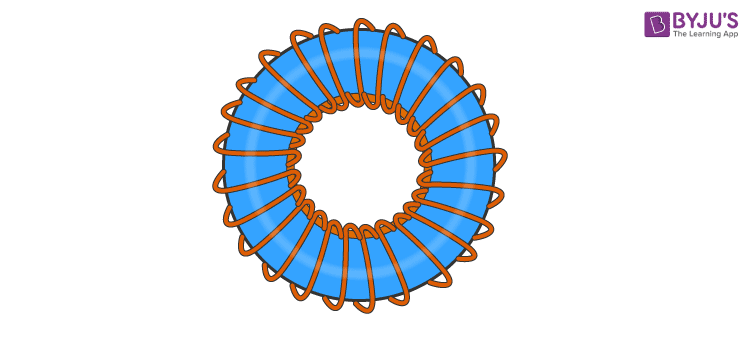## Magnetic Field of Toroid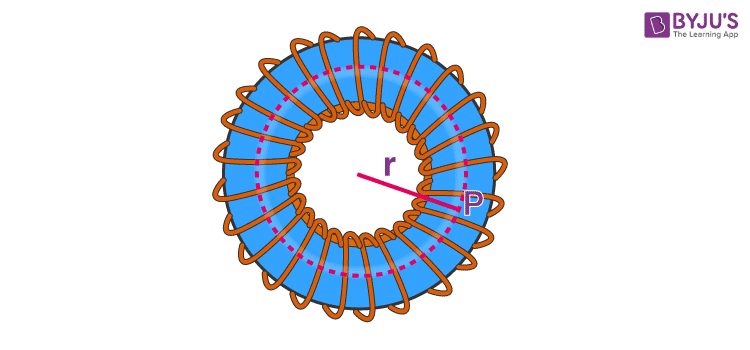The magnetic field of a toroid is calculated by applying Ampere circuit law.

Consider a hollow circular ring with many turns of the current-carrying wire that is wound around it. In the above figure, let the magnetic field, B be present at point P which is inside the toroid. In the above figure, the loop is considered as an amperian loop that forms a circle through point P resulting in concentric circles inside the toroid.

Due to the symmetric field, the magnitude at all the points in the circle is equal, and the field is tangential.

Therefore,

$$\begin{array}{l}\oint B/dl=B\int dl = 2 \pi rB\end{array}$$

If the number of turns in the loop is N, then the net current crossing the area is NI.

Where I is the current in the toroid.

Using Ampere’s law,

$$\begin{array}{l}\oint B.dl=\mu _{o}NI\end{array}$$
$$\begin{array}{l}2 \pi rB =\mu _{o}NI\end{array}$$
$$\begin{array}{l}B=\frac{\mu _{o}NI}{2 \pi r}\end{array}$$

Therefore, we can conclude that the magnetic field B varies and is not uniform over the cross-section.

## Formula of Toroid

The formula of the toroid is used for the calculation of the number of turns in a toroidal coil.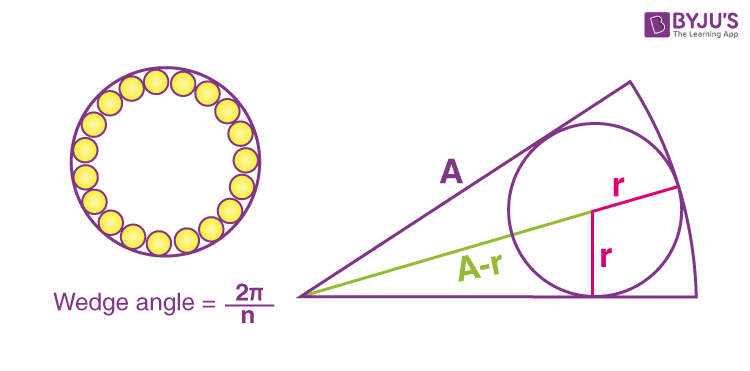The above figure is a pictorial representation of the cross-sectional view of the inner radius of the toroid and the wire. Let,

• A be the inner radius of the toroid
• r be the radius of the wire
• n be the number of loops

The wedge angle is given as:

 $$\begin{array}{l}Wedge\;angle=\frac{2 \pi}{n}\;radians\end{array}$$

The relation between A, r, and n is given in the form of an equation:

 $$\begin{array}{l}sin\frac{\pi}{n}=\frac{r}{A-r}\;radians\end{array}$$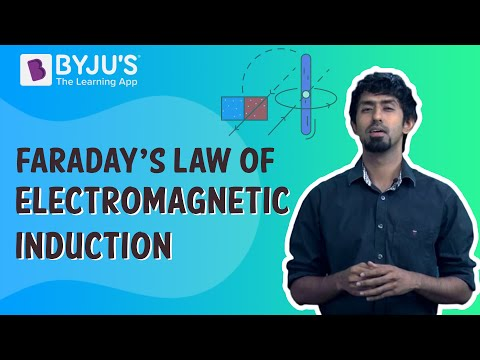### Difference between Solenoid and Toroid

The working principle of both solenoid and toroid is based on electromagnetism. This is one of the similarities between the two. However, there is a difference between solenoid and toroid as for a given toroidal coil, the amount of electric current is more than the solenoidal coil such that they are of the same size.

 Solenoid Toroid The shape of the solenoid is cylindrical The shape of the toroid is circular The formation of the magnetic field is outside the solenoid The formation of the magnetic field is within the toroid The magnetic field inside the solenoid is uniform The magnetic field inside the toroid is non-uniform The magnetic field is given as: B = μonl The magnetic field is given as: B = μNl/2πr

### Similarities between Solenoid and Toroid

• Both are designed on the principle of electromagnetism.
• Both behave like electromagnetic material when an electric current is applied.
• The magnetic field within the toroid and due to the solenoid is the same.

### Applications of Toroid

• It is used in telecommunication.
• Modern medical equipment has toroid in it.
• It finds application in the design of musical instruments.

The below video explains Ampere’s circuital law: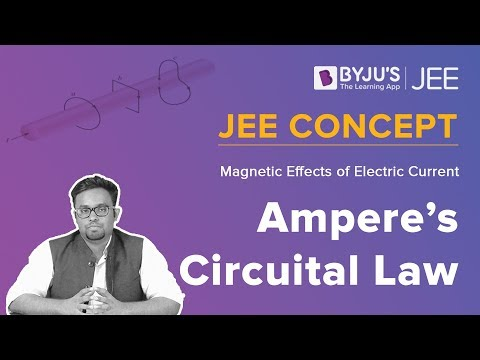## Frequently Asked Questions – FAQs

Q1

### What is Ampere’s circuital law?

Ampere’s circuital law states that the line integral of the magnetic field B around the closed-loop is equal to the product of total current through the loop and permeability, μo.

Q2

### What is the magnetic field in the empty space enclosed by the toroid of radius R?

We know that the magnetic field is confined only to the inside body of the toroid. This is in the form of concentric magnetic lines of force. Therefore, any point in the empty space surrounded by a toroid will have a magnetic field, B equal to zero. The magnetic field is zero because the net current in this space is zero. Thus, the magnetic moment of the toroid is zero.

Q3

### Name the factor on which the magnetic field of a current-carrying toroid is independent of.

The magnetic field of a current-carrying toroid is independent of the radius. This is because the magnetic field of the toroid is given as B = μonI where n is the number of turns, I is the electric current, and μo is the permeability.

Q4

### Name the laws that give the direction of induced emf.

Lenz’s law gives the direction of induced emf.
Lenz’s law states that the direction of induced current in a given magnetic field is such that it opposes the induced change due to a change in the magnetic field.

Q5

### What happens in a current-carrying conductor when the electric field is varied?

When there is variation in the electric field of a current-carrying conductor, there is a generation of magnetic fields.